Home > ACC7 > Chapter cc38 > Lesson cc38.1.2 > Problem8-20

8-20.
1. Jack noticed a sale on his favorite model of All-Terrain Vehicle (ATV). The salesperson said the company was cutting the price for that weekend, so Jack got an 8% discount. Homework Help ✎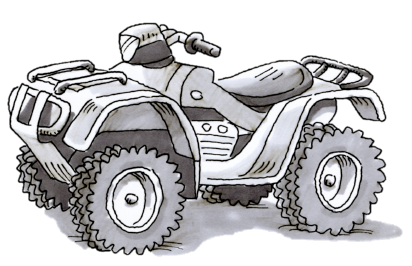1. If the usual price of the ATV was $4298, estimate how much the discount will be. Explain your reasoning. 2. Calculate the amount Jack did pay with the 8% discount. 3. If the store sold three of the ATVs that weekend, how much money was saved by the three customers combined?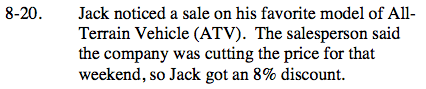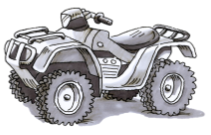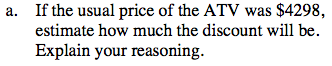Round the ATV price to$4300 in order to make the percent easier to approximate.

Since we can find that 10% of $4300 is$430, we can also determine that 5% of $4300 would be$215. 8% is roughly in the middle of 10% and 5%. What is your estimation?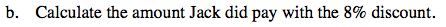Find 8% of $4298, then subtract that value from$4298 to find how much Jack paid.

4298 − 343.84 =

4298(.08) = 343.84

$3954.16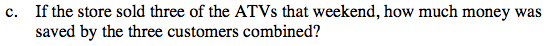For each ATV, the original price was$4298. Based on part (b), it costs \$3954.16 after the 8% discount. How much did Jack save on one ATV? Now multiply that number by 3.